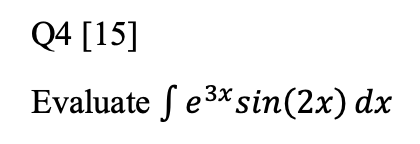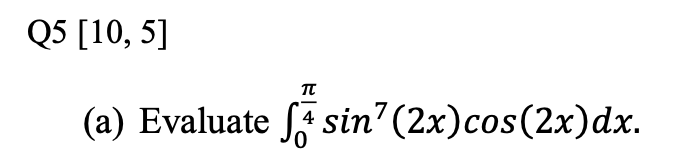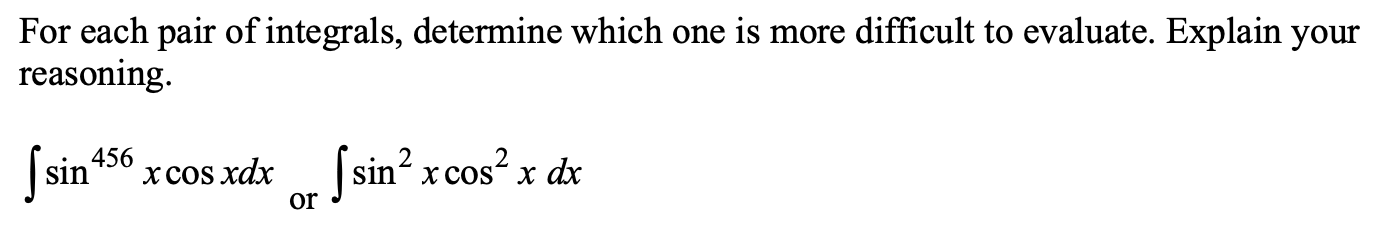Home / Expert Answers / Calculus / q5-part-b-q4-15-evaluate-e3xsin-2x-dx-q5-10-5-a-evaluate-04-pa365

# (Solved): Q5 PART B Q4  Evaluate e3xsin(2x)dx Q5[10,5] (a) Evaluate 04 ...Q5 PART BQ4  Evaluate (a) Evaluate . For each pair of integrals, determine which one is more difficult to evaluate. Explain your reasoning.

We have an Answer from Expert

Sol-4.

To evaluate: the value of the integral   ?

Reorder    and   .

Integrate by parts using the formula   , where    and   .

Since    is constant with respect to   , move    out of the integral.

Combine fractions.

Integrate by parts using the formula   , where    and   .

Since    is constant with respect to   , move    out of the integral.

Simplify terms.

Solving for   , we find that    =   .